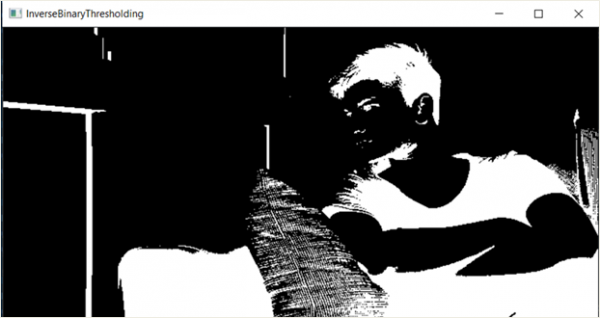# Performing inverse binary thresholding on an image using OpenCV

In this program, we will perform inverse binary thresholding on an image using openCV. Thresholding is a process in which the value of each pixel is changed in relation to a threshold value.

The pixel is given a certain value if it is less than the threshold and some other value if it is greater than the threshold. In inverse binary thresholding, if the value of the pixel is less than the threshold, it will be given a maximum value i.e. white. If it is greater than the threshold, it will be assigned 0, i.e., black.

## Original Image## Algorithm

Step 1: Import cv2.
Step 2: Define threshold and max_val.
Step 3: Pass these parameters in the cv2.threshold value and specify the type of thresholding you want to do.
Step 4: Display the output.

## Example Code

import cv2

threshold_value = 120
max_val = 255

ret, image = cv2.threshold(image, threshold_value, max_val, cv2.THRESH_BINARY_INV)
cv2.imshow('InverseBinaryThresholding', image)

## Output## Explanation

The ret variable in the program simply returns the threshold value. For any pixels having value smaller than the threshold value, they are replaced by max_val, i.e., 255.

Updated on: 17-Mar-2021

906 Views